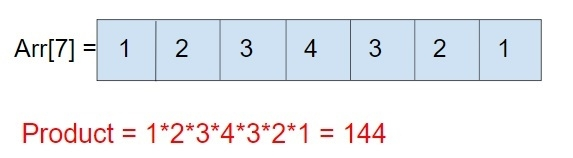# C Program for product of array

Given an array arr[n] of n number of elements, the task is to find the product of all the elements of that array.

Like we have an array arr of 7 elements so its product will be like## Example

Input: arr[] = { 10, 20, 3, 4, 8 }
Output: 19200
Explanation: 10 x 20 x 3 x 4 x 8 = 19200
Input: arr[] = { 1, 2, 3, 4, 3, 2, 1 }
Output: 144

The approach used below is as follows

• Take array input.
• Find its size.
• Iterate the array and Multiply each element of that array
• Show result

## Algorithm

Start
In function int prod_mat(int arr[], int n)
Step 1-> Declare and initialize result = 1
Step 2-> Loop for i = 0 and i < n and i++
result = result * arr[i];
Step 3-> Return result
int main()
Step 1-> Declare an array arr[]
step 2-> Declare a variable for size of array
Step 3-> Print the result

## Example

Live Demo

#include <stdio.h>
int prod_arr(int arr[], int n) {
int result = 1;
//Wil multiply each element and store it in result
for (int i = 0; i < n; i++)
result = result * arr[i];
return result;
}
int main() {
int arr[] = { 10, 20, 3, 4, 8 };
int n = sizeof(arr) / sizeof(arr);
printf("%d", prod_arr(arr, n));
return 0;
}

## Output

If run the above code it will generate the following output −

19200